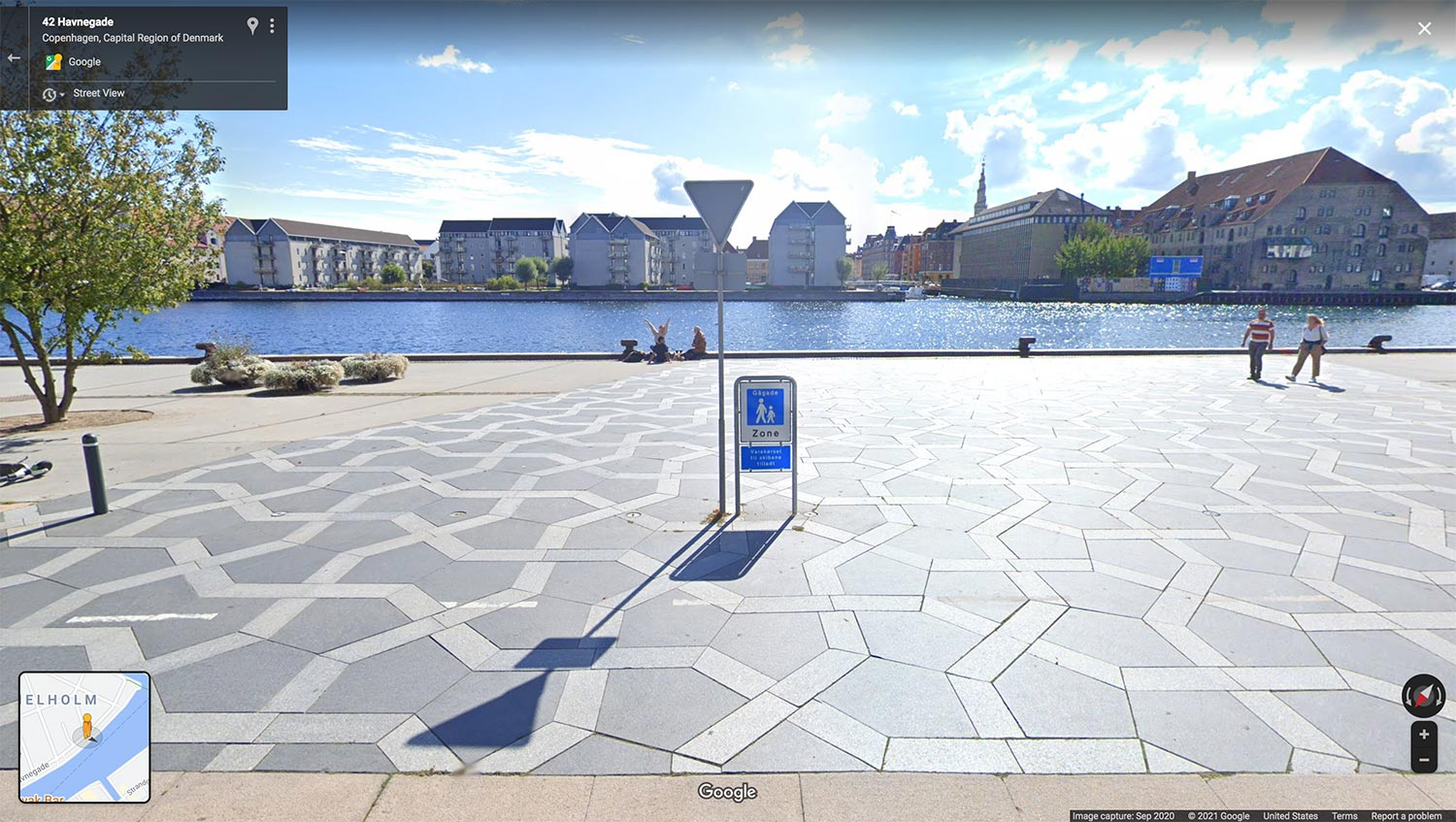As is often the case, not all of these are links; they’re copies of posts that I made over on my Mastodon account because they were too short to make full posts here.

• Borromean rings ($$\mathbb{M}$$), now a Good Article on Wikipedia. You can’t make the Borromean rings with geometric circles: as Tverberg observed, an inversion would make one of them a line. But then each of the other two circles would span an angle less than $$\pi$$ as viewed from the line, leaving an unspanned direction along which the line could escape to infinity, contradicting the inseparability of the rings.

The same proof shows that Brunnian links cannot be made from four geometric circles. But the original proof that Borromean rings are not circular, by Freedman and Skora using 4d hyperbolic geometry, applies more generally to any Brunnian link no matter how many components it has.

• Squares can be divided into $$45^\circ$$–$$60^\circ$$–$$75^\circ$$ triangles ($$\mathbb{M}$$), as Ed Pegg posts on twitter. This is an interesting variant on something I looked at a long time ago, triangulating the square to minimize the maximum angle. But Pegg’s subdivision is not edge-to-edge. And the triangles on my page are not all similar. Do squares have edge-to-edge triangulations by similar acute triangles?

• Science joins other publishers in making it easy for authors to change their names on past publications ($$\mathbb{M}$$, via). Transgender scientists made this change necessary but I think it benefits all of us. I’ve seen plenty of name changes in the literature for other reasons (marriage, divorce, escaping prejudice, …) and making it easier to find your old papers is generally a good thing.

• Cairo in Denmark? ($$\mathbb{M}$$). This is a screenshot from Google Maps of Havnepromenade in Copenhagen, where it meets the end of Cort Adelers Gade. I’m sad to have missed this spot when I was in Copenhagen a couple years ago; it’s a nice example of a Cairo pentagonal tiling.• My latest illustration trick: make complex non-Boolean combinations of shapes by overlaying simple shapes, exploding the overlay into simple regions, and unioning the regions into the shapes I want ($$\mathbb{M}$$). Exploding and unioning are single clicks in Illustrator’s pathfinder tool. I used two levels of this technique to make this diagram of a Brunnian link: once to make the shape of a single component, and again to make the over-under relation between components.• Real induction ($$\mathbb{M}$$). An induction principle for proving statements about all real numbers in intervals.

• Recamán Sequence ($$\mathbb{M}$$). Linocut art by Josh Millard in a style drawn from a Numberphile video.

• Quanta surveys recent developments in fast matrix multiplication ($$\mathbb{M}$$), including a paper from last SODA by Josh Alman and Virginia Williams improving the exponent from 2.3728639 to 2.3728596. Known barriers to the widely-expressed hope that the exponent can be reduced to 2 (see the SODA paper’s introduction) are, I think too specific to be convincing. On the other hand at this rate there’s still a long way from here to 2…

• Printing algebraic numbers ($$\mathbb{M}$$, via). So your code can handle symbolic representations of exact algebraic numbers rather than just approximating everything as complex/float. How do you get those representations out to human users in a readable way? From ‪Fredrik Johansson‬, long-time developer of open symbolic algebra and exact real arithmetic software including SymPy and Calcium.

• Fermat’s right triangle theorem ($$\mathbb{M}$$), now a Good Article on Wikipedia. As Fibonacci observed and Fermat proved (as his only surviving proof), integer right triangles cannot have an area that is a perfect square. One corollary of this is the exponent-4 case of Fermat’s Last Theorem.

• Tom Harris asks: What’s your favorite OEIS sequence? Mine for this week at least is the Moser–De Bruijn sequence, OEIS A000695.

• The most recent image of me on my site was from 2009 and getting pretty out-of-date. So I took a screenshot of myself as I look these days on Zoom ($$\mathbb{M}$$). This is far from the first time I’ve let my hair go at least this long, but I’m still looking forward to a time when I will feel more comfortable getting a haircut. It occurs to me that there has been a reversal: now, instead of showing off rebelliousness, I am visibly conforming to safety rules.• Weird probability distributions on dyadic rationals from a simple averaging process ($$\mathbb{M}$$). Start with the two-element multiset $$\{0,1\}$$, repeatedly draw two-element samples (without replacement) from the multiset and include the average into the multiset. The result tends to cluster around some value, not the same value every time but itself drawn from a bimodal distribution, with the clustering sort of looking Cauchy but not quite. Interesting new MathOverflow question.

• How many hyperplanes does it take to slice all the edges of an -dimensional hypercube ($$\mathbb{M}$$)? Two if you can skim the ends of the edges or include entire edges in hyperplanes but more to really slice them. The obvious answer of axis-parallel cuts can be improved to $$5n/6$$, and Lance Fortnow reports that the newest lower bound is $$\Omega(n^{0.57})$$, by Gal Yehuda and Amir Yehudayoff, improving their own new preprint.

• A list of current blogs on computer theory and related math ($$\mathbb{M}$$), from Gödel’s Lost Letter and P=NP.